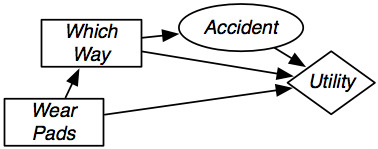9.2.1 Single-Stage Decision Networks

The decision tree is a state-based representation because the worlds correspond to the resulting state. It is, however, often more natural and more efficient to represent and reason in terms of features, represented as variables.

A single-stage decision network is an extension of a belief network that has three kinds of nodes:

• Decision nodes, drawn as rectangles, represent decision variables. The agent gets to choose a value for each decision variable. Where there are multiple decision variables, we assume there is a total ordering of the decision nodes, and the decision nodes before a decision node D in the total ordering are the parents of D.
• Chance nodes, drawn as ovals, represent random variables. These are the same as the nodes in a belief network. Each chance node has an associated domain and a conditional probability of the variable, given its parents. As in a belief network, the parents of a chance node represent conditional dependence: a variable is independent of its non-descendants, given its parents. In a decision network, both chance nodes and decision nodes can be parents of a chance node.
• A utility node, drawn as a diamond, represents the utility. The parents of the utility node are the variables on which the utility depends. Both chance nodes and decision nodes can be parents of the utility node.

Each chance variable and each decision variable has an associated domain. There is no domain associated with the utility node. Whereas the chance nodes represent random variables and the decision nodes represent decision variables, there are no associated utility variables. The utility provides a function of its parents.

Associated with a decision network is a conditional probability for each chance node given its parents (as in a belief network) and a representation of the utility as a function of the utility node's parents. In the specification of the network, there are no tables associated with the decision nodes.Which Way Accident Value short true 0.2 short false 0.8 long true 0.01 long false 0.99
 Wear Pads Which Way Accident Utility true short true 35 true short false 95 true long true 30 true long false 75 false short true 3 false short false 100 false long true 0 false long false 80
Figure 9.5: Single-stage decision network for the delivery robot

Example 9.8: Figure 9.5 gives a decision network representation of Example 9.4. There are two decisions to be made: which way to go and whether to wear padding. Whether the agent has an accident only depends on which way they go. The utility depends on all three variables.

This network requires two factors: a factor representing the conditional probability, P(Accident|WhichWay), and a factor representing the utility as a function of Which Way, Accident, and Wear Pads. Tables for these factors are shown in Figure 9.5.

A policy for a single-stage decision network is an assignment of a value to each decision variable. Each policy has an expected utility, which is the conditional expected value of the utility conditioned on the policy. An optimal policy is a policy whose expected utility is maximal. That is, it is a policy such that no other policy has a higher expected utility.

1: Procedure OptimizeSSDN(DN)
2:           Inputs
3:                     DN a single stage decision network
4:           Output
5:                     An optimal policy and the expected utility of that policy.
6:           Prune all nodes that are not ancestors of the utility node.
7:           Sum out all chance nodes.
8:           - at this stage there is a single factor F that was derived from utility
9:           Let v be the maximum value in F
10:           Let d be an assignment that gives the maximum value
11:           return d,v
Figure 9.6: Variable elimination for a single-stage decision network

Figure 9.6 shows how variable elimination can be used to find an optimal policy in a single-stage decision network. After pruning irrelevant nodes and summing out all random variables, there will be a single factor that represents the expected utility for each combination of decision variables. This factor does not have to be a factor on all of the decision variables; however, those decision variables that are not included are not relevant to the decision.

Example 9.9: Consider running OptimizeSSDN on the decision network of Figure 9.5. No nodes can be pruned, so it sums out the only random variable, Accident. To do this, it multiplies both factors because they both contain Accident, and sums out Accident, giving the following factor:
 Wear Pads Which Way Value true short 0.2* 35+ 0.8*95=83 true long 0.01*30+0.99*75=74.55 false short 0.2*3+0.8*100=80.6 false long 0.01*0+0.99*80=79.2

Thus, the policy with the maximum value - the optimal policy - is to take the short way and wear pads, with an expected utility of 83.